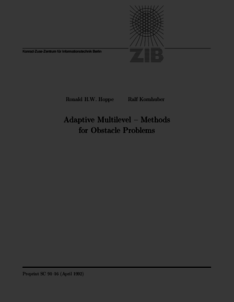Repository: Freie Universität Berlin, Math Department

Adaptive multilevel methods for obstacle problems

Hoppe, R. H. W. and Kornhuber, R. (1994) Adaptive multilevel methods for obstacle problems. SIAM Journal on Numerical Analysis, 31 (2). pp. 301-323. ISSN 0036-1429Preview

339kB

Official URL: http://dx.doi.org/10.1137/0731016

Abstract

The authors consider the discretization of obstacle problems for second-order elliptic differential operators by piecewise linear finite elements. Assuming that the discrete problems are reduced to a sequence of linear problems by suitable active set strategies, the linear problems are solved iteratively by preconditioned conjugate gradient iterations. The proposed preconditioners are treated theoretically as abstract additive Schwarz methods and are implemented as truncated hierarchical basis preconditioners. To allow for local mesh refinement semilocal and local a posteriors error estimates are derived, providing lower and upper estimates for the discretization error. The theoretical results are illustrated by numerical computations.

Item Type: Article Obstacle problems, adaptive finite element methods, multilevel preconditioning, a posteriors error estimates Mathematical and Computer Sciences > Mathematics > Numerical Analysis Department of Mathematics and Computer Science > Institute of Mathematics 1913 Ekaterina Engel 21 Jun 2016 20:22 03 Mar 2017 14:42

Repository Staff Only: item control page